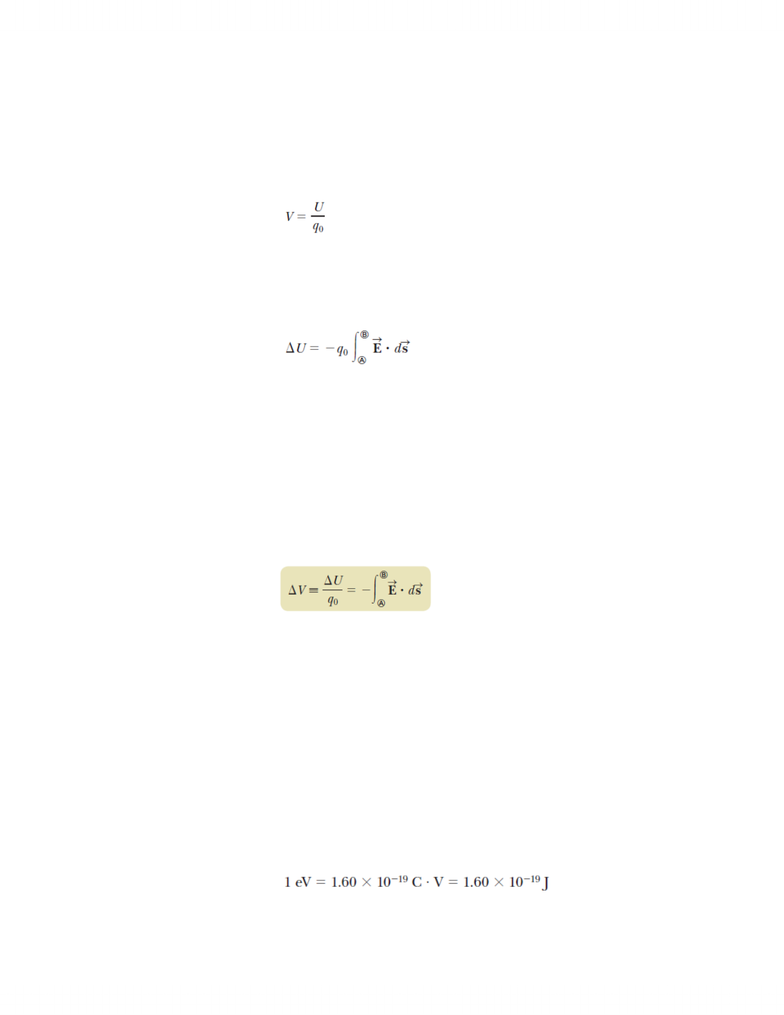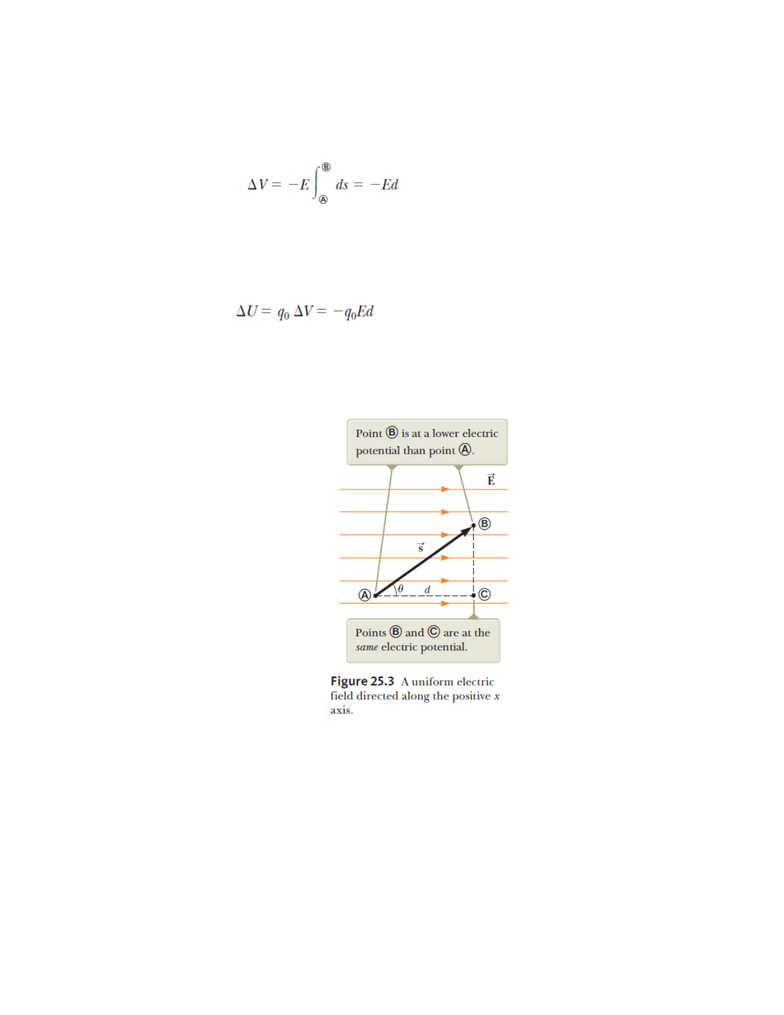Textbook Notes (280,000)
CA (170,000)
McGill (5,000)
Physics (60)
Chapter 25

# PHYS 142 Chapter Notes - Chapter 25: Test Particle, Robert Andrews Millikan, Electric Potential Energy

Department
Physics
Course Code
PHYS 142
Professor
Yoichi Miyahara
Chapter
25

This preview shows pages 1-3. to view the full 14 pages of the document.PHYS 142 Chapter 25 SmartNotes
25.1: Electric Potential and Potential Difference
o Electric Potential
Definition
o Scalar quantity
o SI Unit
Volt (V) = 1 J/C
Change in the Electric Potential Energy of a System
Note:
We often take the value of the electric potential to be zero
at come convenient point in an electric field.
o Potential Difference
Definition
  
 
o SI Unit
Volt (V) = 1 J/C
Potential Difference between Two Points
Note:
Potential Difference is not the same as Potential Energy.
Potential difference between A and B exists solely because a
source charge and depends on the source charge
distribution. For the potential energy to exist, we must have
a system of two or more charges. The potential energy
belongs to the system and changes only if a charge is
moved relative to the rest of the system.
o Electron Volt (eV)
Defined as the energy a charge-field system gains or loses when a
charge of magnitude
e
is moved through a potential difference of 1
V.

Only pages 1-3 are available for preview. Some parts have been intentionally blurred.o Electric Field can also be expressed in Volts/Meter (1 N/C = 1
V/m)

Only pages 1-3 are available for preview. Some parts have been intentionally blurred.25.2: Potential Difference in a Uniform Electric Field
o Potential Difference between two points in a uniform electric
field
point B is lower than point A.
Electric field lines always point in the direction of
decreasing electric potential.
In a system consisting of a positive charge and an electric
field, the electric potential energy of the system decreases
when the charge moves in the direction of the field
(Negative Work)
o
A system consisting of a negative charge and an electric field
gains electric potential energy when the charge moves in the
direction of the field. It accelerates in the opposite direction
of the field. (Positive Work)